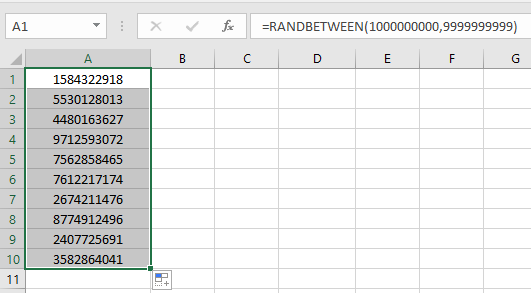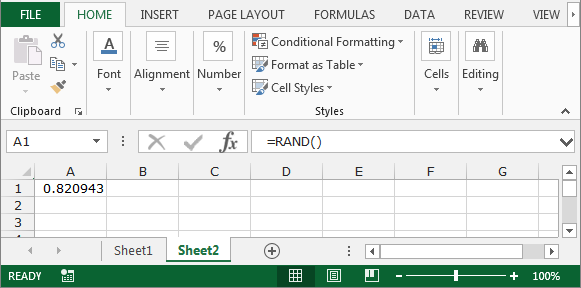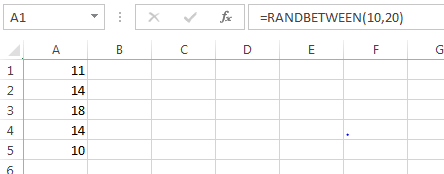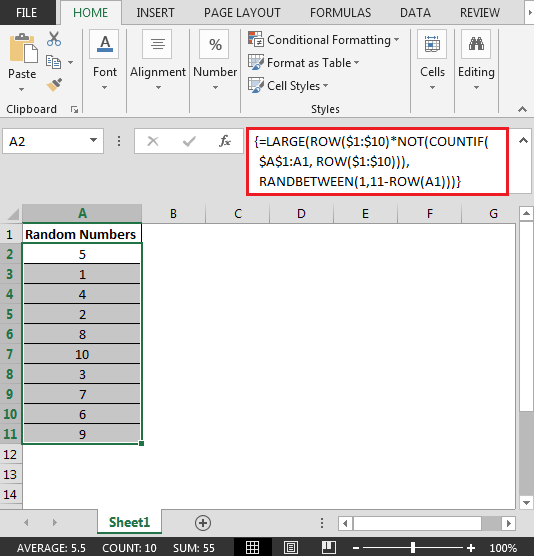# Random Number Generation in Microsoft ExcelIn this article, we will learn the random number generator in Microsoft Excel. To generate the random number, we will use the “RAND” and “RANDBETWEEN” function.

Rand function is used to returns the random number greater than or equal to 0 and less than 1, and also it recalculates all time when we do any activity in the sheet.

Syntax of “RAND” function:         =RAND()

For example:-

• Select the cell A1 and write the formula.
• =RAND(), press Enter.
• The function can return any random numberNote:- Random numbers change every time a cell on the sheet is calculated.

RANDBETWEEN:- This function is used to  extract a random integer number between the two specified (bottom & top) numbers. A new random integer number gets generated every time the worksheet is calculated.

Syntax of RANDBETWEEN:-  =RANDBETWEEN(bottom,top)

• Select the cell A1 and write the formula.
• =RANDBETWEEN(10,20), and press Enter.
• Drag it with the mouse till the cell A5.
• The function will return the random number in between 10, 20.This is all about how we can produce the random numbers by using the formula Rand and Randbetween in Microsoft Excel.

Let’s take an example on array of random numbers without duplicates

In case you want to generate random number in Excel within a specified upper & lower limit without VBA, then you will have to generate Random numbers with Excel Randbetween function. In this article, let’s discuss how to apply this function.

Question: I want to create a list of random numbers between 1 to 10 in a column without repetition.

• In cell A2, the formula is
• {=LARGE(ROW(\$1:\$10)*NOT(COUNTIF(\$A\$1:A1, ROW(\$1:\$10))), RANDBETWEEN(1,11-ROW(A1)))}
• Copy the formula in range A3:A11

Note: This is an array formula. Use CTRL + SHIFT + ENTER keys together• The above formula will generate a list of unique random numbers from 1 to 10.

In this way we can use RAND & RANDBETWEEN functions in excel.

Another way in Excel 2016

RANDBETWEEN function stands for Random number between the 2 numbers. To  get the same, follow the below steps.

RANDBETWEEN function returns the random numbers between any two numbers.

Syntax:

 =RANDBETWEEN ( bottom, top )

Bottom : The smaller of the 2 numbers.

Top : The larger of the 2 numbers.

As we know a phone number have the following condition:

1. It has 10 digits
2. Cannot starts with 0

So the smallest possible number comes out to be 1000000000 and the largest possible number comes out to be  9999999999

So use the formula shown below to generate phone numbers

Use the Formula:

 = RANDBETWEEN ( 1000000000 , 9999999999 )

1000000000 : smaller one

9999999999 : larger oneJust enter the formula once and repeat results using the drag down option in excel as shown in the above snapshot.

As you can see, you can get the random phone numbers in Excel.

Another ways to pick random values from given list

For this, we can opt for any of the two methods mentioned below. One method is using CHOOSE function. In this We need to input all individual values from the list to the function. You can use this formula for when number values in the list are less.

Formula using CHOOSE function:

 = CHOOSE ( RANDBETWEEN ( 1 , n) , "value1" , "value2" , "value3",,,"value n" )

n : number of values in list

value1 , value2 , value3 , till value n : individual values from the list. These values can be input either using the cell reference or using directly with quotes or using the cell reference.

Another method is using the INDEX function where we will feed a random number as index which will result in the corresponding value at the index.

Formula using INDEX function:

 =INDEX(data, RANDBETWEEN (1,ROWS (data)),col_index)

data : data array can be a list or table.

1 : start index

col_index : column index to point out the list in table.

Notes :

1. The function returns an error, if the first number argument ( bottom ) is larger than the second number argument ( top ).
2. The function returns error, if the argument to the function is non - numeric.

Hope this article about Random Numbers Generator without Repeat Numbers using VBA in Microsoft Excel is explanatory. Find more articles on generating random numbers or date values here. If you liked our blogs, share it with your fristarts on Facebook. And also you can follow us on Twitter and Facebook. We would love to hear from you, do let us know how we can improve, complement or innovate our work and make it better for you. Write us at info@exceltip.comRelated Articles :

Excel VBA Variable Scope : In all the programming languages, we have variable access specifiers that define from where a defined variable can be accessed. Excel VBA is no Exception. VBA too has scope specifiers.

All About Excel Named Ranges : excel ranges that are tagged with names are easy to use in excel formulas. Learn all about it here.

Relative and Absolute Reference in Excel : Understanding of Relative and Absolute Reference in Excel is very important to work effectively on Excel. Relative and Absolute referencing of cells and ranges.

Generate Random Phone Numbers : Generate random 10 digti numbers using the RANDBETWEEN formula in Excel

Get Random number From Fixed Options : Generate random numbers form the list having criteria in Excel.

Get Random numbers between two numbers : RANDBETWEEN function generator number between the two given numbers in Excel.

Excel Random Selection: How to Get Random Sample From a Dataset : Use the random samples in Excel for the explained examples here.

How to use the RANDBETWEEN Function in Excel : Excel RANDBETWEEN function returns a number between two given numbers in Excel.

Popular Articles :

50 Excel Shortcuts to Increase Your Productivity : Get faster at your task. These 50 shortcuts will make you work even faster on Excel.

How to use the IF Function in Excel : The IF statement in Excel checks the condition and returns a specific value if the condition is TRUE or returns another specific value if FALSE.

How to use the VLOOKUP Function in Excel : This is one of the most used and popular functions of excel that is used to lookup value from different ranges and sheets.

How to Use SUMIF Function in Excel : This is another dashboard essential function. This helps you sum up values on specific conditions.

How to use the COUNTIF Function in Excel : Count values with conditions using this amazing function. You don't need to filter your data to count specific values. Countif function is essential to prepare your dashboard.

Terms and Conditions of use

The applications/code on this site are distributed as is and without warranties or liability. In no event shall the owner of the copyrights, or the authors of the applications/code be liable for any loss of profit, any problems or any damage resulting from the use or evaluation of the applications/code.